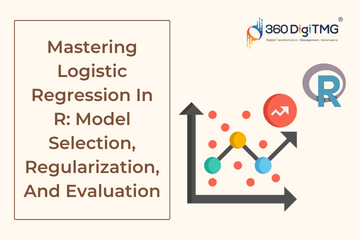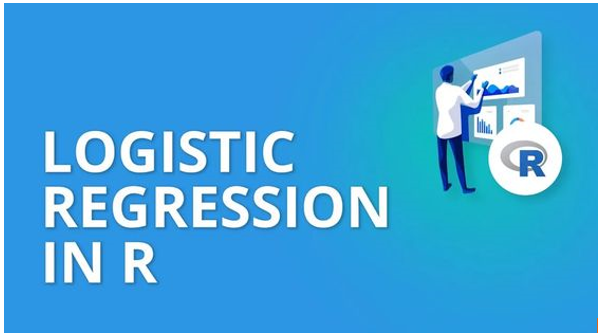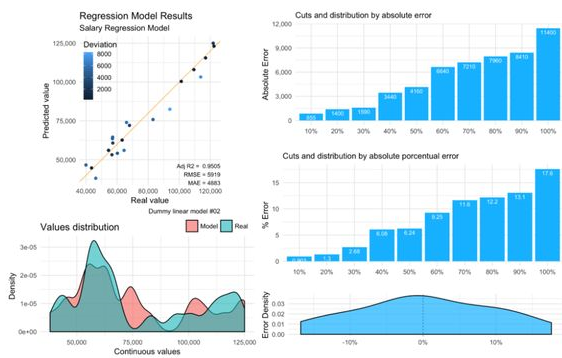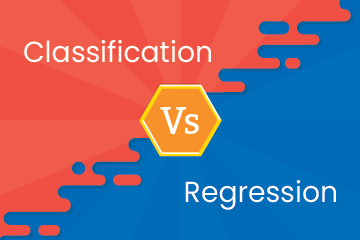Home / Blog / Data Science / Mastering Logistic Regression In R: Techniques For Model Selection, Regularization, And Evaluation

# Mastering Logistic Regression In R: Techniques For Model Selection, Regularization, And Evaluation

• March 01, 2023
• 3340
• 89### Meet the Author : Mr. Bharani Kumar

Bharani Kumar Depuru is a well known IT personality from Hyderabad. He is the Founder and Director of Innodatatics Pvt Ltd and 360DigiTMG. Bharani Kumar is an IIT and ISB alumni with more than 17 years of experience, he held prominent positions in the IT elites like HSBC, ITC Infotech, Infosys, and Deloitte. He is a prevalent IT consultant specializing in Industrial Revolution 4.0 implementation, Data Analytics practice setup, Artificial Intelligence, Big Data Analytics, Industrial IoT, Business Intelligence and Business Management. Bharani Kumar is also the chief trainer at 360DigiTMG with more than Ten years of experience and has been making the IT transition journey easy for his students. 360DigiTMG is at the forefront of delivering quality education, thereby bridging the gap between academia and industry.

Logistic Regression is a popular statistical method used to model binary outcomes. It is frequently used to comprehend the connection between a collection of independent variables and a binary dependent variable in disciplines like medicine, economics, and the social sciences. This article will explore Logistic Regression and how to implement it in R.Logistic Regression is a popular statistical technique for predicting binary outcomes. R is a powerful programming language for data science and machine learning, and it provides several packages you can use to implement logistic Regression. This article covers the fundamentals of Logistic Regression in R, including how to fit a logistic regression model and how to assess the effectiveness of a logistic regression model. In addition, here are some best practices for using Logistic Regression in R. With this knowledge, you can now start using logistic Regression to analyze your data and make predictions about binary outcomes.

### What is Logistic Regression?

A statistical method called logistic Regression is used to predict binary outcomes. A binary outcome is an outcome that can only take one of two possible values, such as "yes" or "no," "pass" or "fail," "positive" or "negative," etc. One can use the Logistic Regression model to check the probability of the binary outcome as a function of one or more variables that are independent.

The logistic regression model assumes that the probability of the binary outcome is a function of a linear combination of the independent variables. The logistic function transforms the linear combination into a probability value between 0 and 1.

### Learn the core concepts of Data Science Course video on YouTube:

The logistic function is defined as follows:

p = 1 / (1 + e^(-z))

where p is the probability of the binary outcome, z is the linear combination of the independent variables, and e is the base of the natural logarithm.

In logistic Regression, we estimate the coefficients of the independent variables in the linear combination to maximize the likelihood of the observed binary outcomes. Then, you can use the estimated coefficients to calculate the probability of the binary output for a given set of independent variables.

### Implementing Logistic Regression in R

For data science and machine learning, R is a well-liked programming language. It has several packages that can be used to implement logistic Regression. The most commonly used package is the "glm" package. In this, "glm" means Generalized Linear Models, and you can use it to fit various models, including logistic Regression. Here's how you can implement logistic Regression in R:

You can implement the logistic Regression using the glm() function in R. The glm() function stands for the Generalized Linear Model, a general framework for modeling various outcomes, including binary outcomes.

Step 1: Load the Data The first step is loading of the data into R. Next, you can use the "read.csv" function to read the data from a CSV file. Once the data is loaded, you can examine the first few rows of the data by using the "head" function.

Step 2: Preprocess the Data The second step is to preprocess the data. It involves converting categorical variables into dummy variables and scaling the numerical variables. You can use the "dplyr" package to accomplish this. The "dplyr" package provides several functions such as "mutate," "select," "filter," and "group_by" that you can use to preprocess the data.

Step 3: Split the Data The third step is to split the data into training and testing sets. You can do it by using the "caret" package. The "caret" package provides the "createDataPartition" function that you can use to split the data into training and testing sets based on a specified proportion.

Step 4: Fit the Logistic Regression Model The final step is to fit the logistic regression model using the "glm" function. The "glm" function takes several arguments, including the formula, data, and family. The formula specifies the dependent variable and the independent variables. The data is preprocessed, and the family is "binomial" for logistic Regression.

Here below is an example of how to implement logistic Regression in R using the glm() function:

rCopy code
# Fit the logistic regression model
model <- glm(outcome ~ x1 + x2 + x3, data = data, family = binomial())
# Print the summary of the model summary(model)

In this example, we have a binary outcome variable called "outcome" and three independent variables called "x1", "x2", and "x3". We use the glm() function to fit the logistic regression model, specifying the formula for the model as "outcome ~ x1 + x2 + x3", the data as "data," and the family as "binomial."

You can use the summary() function to print the model summary, which includes the estimated coefficients, standard errors, z-values, and p-values for each independent variable in the model.### Interpreting the Results of Logistic Regression:

You can interpret the results of logistic Regression in several ways. The following are some crucial considerations for understanding the outcomes of a logistic regression model:

1. Coefficients: The coefficients represent the change in the log odds of the binary outcome for a one-unit increase in the corresponding independent variable. A positive coefficient indicates that the probability of the binary outcome increases with an increase in the corresponding independent variable. In contrast, a negative coefficient indicates that the possibility of the binary outcome decreases with an increase in the corresponding independent variable.

2. Odds Ratio: The odds ratio is the exponential of the coefficient, representing the change in odds of the binary outcome for a one-unit increase in the corresponding independent variable. If the odds ratio is higher than 1, it means that as the corresponding independent variable increases, there is a greater chance that the binary result will occur. An odds ratio of less than 1 suggests that the odds of the binary outcome decrease with an increase in the corresponding independent variable.

3. Goodness of Fit: You can evaluate the logistic regression model's goodness of fit using a variety of metrics, including deviance.

### Regularization in Logistic Regression:

Regularization is a method of adding a penalty term to the loss function to prevent overfitting. For instance, by including a penalty word in the cost function, you can use it to prevent overfitting. There are two types of regularization techniques: L1 regularization and L2 regularization. In L1 regularization, the penalty term is the absolute value of the coefficients, while in L2 regularization, it is the square of the coefficients.

1. Logistic Regression in R: Programming systems like R are some of the most popular ones for data analysis and statistical computing. It provides a wide range of packages for machine learning and data visualization. For example, logistic Regression is a commonly used technique in R for binary classification problems.

To perform logistic Regression in R, we need to follow these steps:

First, import the data: The first step is to import the data into R. The data can be in any format, such as CSV, Excel, or text.

• Data Preprocessing: After importing the data, we need to preprocess it. It includes handling missing values, encoding categorical variables, and scaling the features.
• Split the data: We need to split the data into training and testing sets. You can use the training set to train the model and the testing set to evaluate the model's performance.
• Build the model: We can use R's glm() function to build the logistic regression model. The formula argument specifies the dependent variable and the independent variables.
• Evaluate the model: Using different metrics like accuracy, precision, recall, and F1 score, we can assess the model's success.

### Logistic Regression Variants:

Multinomial Logistic Regression: When there are more than two groups in the dependent variable, you will use the multinomial logistic Regression. It is also known as softmax regression. In this variant, we use the softmax function instead of the sigmoid function to calculate the probabilities of each class.

Ordinal Logistic Regression: Ordinal logistic Regression is used when the dependent variable is ordinal, i.e., it has a natural order. In this variant, we assume that the effect of the independent variables on the dependent variable is proportional across the levels of the ordinal variable.

Logistic Regression with Interaction Effects: When the level of one independent variable affects the level of another independent variable, this is known as an interaction effect. Logistic Regression with interaction effects is used to model such relationships.

Logistic Regression with Regularization: Regularization is used to prevent overfitting in logistic Regression. There are two types of regularization techniques: L1 regularization and L2 regularization. L1 regularization adds a penalty term proportional to the absolute value of the coefficients, while L2 regularization adds a penalty term proportional to the coefficients' square. Regularization helps to simplify the model and avoid overfitting.

Logistic Regression with Penalized Likelihood: The penalized likelihood is another technique used to prevent overfitting in logistic Regression. It adds a penalty term to the likelihood function instead of the loss function.

Logistic Regression with Bayesian Methods: Bayesian methods can be used to perform logistic Regression. In Bayesian logistic Regression, we specify a prior distribution for the coefficients and update it based on the data.1. Model Selection: One of the essential steps in building a logistic regression model is selecting the right set of variables to include in the model. This process can also be called as feature selection. In R, there are different methods to perform feature selection, such as Forward Selection, Backward Selection, and Stepwise Selection.

Forward Selection involves starting with a model containing no predictor variables and then adding variables based on their significance. Backward Selection is exactly the opposite of Forward Selection. It consists of creating a model containing all predictor variables and then removing them one by one based on their significance. Finally, stepwise Selection is a combination of Forward and Backward Selection.

2. Regularization Techniques: Regularization techniques are used to prevent the overfitting of the model on the training data. Regularization methods add a penalty term to the objective function the model tries to optimize. Two standard regularization techniques used in logistic Regression are L1 regularization (also called Lasso regularization) and L2 regularization (also called Ridge regularization).

L1 regularization adds a penalty term proportional to the model coefficients' absolute value. This method is proper when the dataset contains many predictor variables; some need to be revised or revised. L2 regularization adds a penalty term proportional to the square of the model coefficients. Again, this method is useful when the dataset contains many predictor variables, all potentially relevant.

3. Model Evaluation: Once the logistic regression model is built, it must be evaluated to check its performance. One commonly used metric to assess the performance of a binary classification model like logistic Regression is Receiver Operating Characteristic (ROC) curve. The ROC curve plots between True Positive Rate (TPR) and False Positive Rate (FPR).

Another commonly used metric is the area under the ROC curve (AUC). AUC is a single scalar value to measure the model's overall performance. An AUC value of 0.5 indicates a random guess, while an AUC value of 1 indicates a perfect classifier. You can use the "pROC" package to calculate the ROC curve and AUC in R.

### Applications of Logistic Regression:

Logistic Regression is widely used in various applications such as:

1. Fraud Detection: Logistic Regression is used to identify fraudulent transactions.

2. Credit Scoring: Logistic Regression is used in credit scoring to predict the likelihood of default.

3. Medical Diagnosis: Logistic Regression is used to predict the likelihood of disease.

4. Marketing Analytics: Logistic Regression is used to predict the likelihood of a customer purchasing.

5. Sentiment Analysis: Logistic Regression is used in sentiment analysis to classify the sentiment of text data.

6. Image Classification: Logistic Regression is used to classify images into different categories.

### How to Evaluate the Performance of a Logistic Regression Model:

Once you have fit a logistic regression model in R, it is essential to evaluate its performance. You can use several metrics to assess the performance of a logistic regression model. Here are few of the most commonly used metrics are

• Confusion Matrix: It is a table used to evaluate a classification model's performance. It shows the number of true positives, false positives, and false negatives.

• Accuracy: Accuracy is the percentage of instances that are properly classified. You can determine it by dividing the total number of cases by the number of samples that were properly classified.

• Precision: : Precision is the proportion of correctly predicted positive instances out of all predicted positive examples. You can determine it by dividing the total number of true positives by the true and false positives sum.

• Recall: Recall is the proportion of correctly predicted positive instances out of all positive ones. You can determine it by dividing the n of true positives (i.e., number) by the true positives and false negatives sum.

• F1 Score: The harmonic mean of recall and precision is known as the F1 score. You can calculate it as 2 * (precision * recall) / (precision + recall).

You can use the "caret" package in R to evaluate the logistic regression model's performance. The "caret" package provides several functions such as "confusionMatrix", "accuracy," "precision," "recall," and "fMeasure" that can be used to calculate these metrics.

### Conclusion:

In conclusion, Logistic Regression is a powerful machine-learning technique used for binary classification problems. You can use it in various applications such as fraud detection, credit scoring, medical diagnosis, marketing analytics, sentiment analysis, and image classification. In this article, we have discussed the basics of logistic Regression, how it works, and how it can be implemented in R. We also discussed various variants of logistic Regression and their applications. With this knowledge, you can build and use your logistic regression models to solve real-world problems.

Do You want more about Classification vs Regression

### Data Analyst Courses in Other Locations